# How to calculate compound interest in Excel: daily, monthly, yearly compounding

The tutorial explains the compound interest formula for Excel and provides examples of how to calculate the future value of the investment at annual, monthly or daily compounding interest rate. You will also find the detailed steps to create your own Excel compound interest calculator.

Compound interest is one of the basic building blocks in banking and one of the most powerful financial forces around that determine the outcome of your investments.

Unless you are an accounting graduate, financial analyst or an experienced investor, it might be a bit difficult to grasp the concept from specialized financial books and manuals. The aim of this article is to make it easy : ) You will also learn how to use a compound interest formula in Excel and create a universal compound interest calculator for your own worksheets.

## What is compound interest?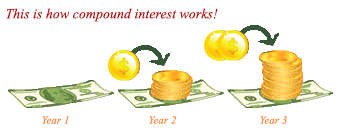In very simple terms, compound interest is the interest earned on interest. More precisely, compound interest is earned on both the initial deposit (principal) and the interest accumulated from previous periods.

Perhaps, it might be easier to start with simple interest that is calculated only on the principal amount. For example, you put \$10 into a bank account. How much will your deposit be worth after one year at an annual interest rate of 7%? The answer is \$10.70 (10 + 10*0.07 = 10.70), and your earned interest is \$0.70.

In case of compound interest, the principal in each time period is different. The bank won't give the earned interest back to you, instead they add it to your principal investment. This increased amount becomes the principal for the next time period (compounding period) and also earns interest. In other words, you earn interest not only on the principal amount, but also on the interest earned in each compounding period.

In our example, in addition to the principal amount of \$10, the earned interest of \$0.70 will also earn interest next year. So, how much will your \$10 deposit be worth after 2 years at the annual interest rate of 7% compounded yearly? The answer is \$11.45 (10.7 + 10.7*0.07 = 11.45) and your earned interest is \$1.45. As you see, at the end of the second year, not only did you earn \$0.70 on the initial \$10 deposit, you also earned \$0.05 on the \$0.70 interest that accumulated in the first year.

There are several ways to calculate compound interest in Excel, and we are going to discuss each one in detail.

## How to calculate compound interest in Excel

Long time investments can be an effective strategy to increase your wealth, and even small deposits can make a big difference over time. The Excel compound interest formulas explained further will help you get the savings strategy to work. Eventually, we are going to make a universal formula that calculates the future value with different compounding periods - daily, weekly, monthly, quarterly, or yearly.

### Calculating annual compound interest in Excel

To understand the idea of compound interest better, let's begin with a very simple example discussed at the beginning of this tutorial and write a formula to calculate annual compound interest in Excel. As you remember, you are investing \$10 at the annual interest rate of 7% and want to know how yearly compounding increases your savings.

#### Annual compound interest - formula 1

An easy and straightforward way to calculate the amount earned with an annual compound interest is using the formula to increase a number by percentage:

`=Amount * (1 + %)`.

In our example, the formula is:

`=A2*(1+\$B2)`

Where A2 is your initial deposit and B2 is the annual interest rate. Please pay attention that we fix the reference to column B by using the \$ sign.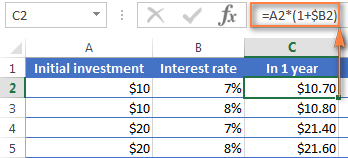As you remember, 1% is one part of a hundred, i.e. 0.01, so 7% is 0.07, and this is how percentages are actually stored in Excel. Keeping this in mind, you can verify the result returned by the formula by performing a simple calculation of 10*(1+0.07) or 10*1.07 and make sure that your balance after 1 year will be \$10.70 indeed.

And now, let's calculate the balance after 2 years. So, how much will your \$10 deposit be worth in two years' time at an annual interest rate of 7%? The answer is \$11.45 and you can get it by copying the same formula to column D.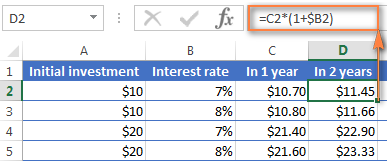To calculate how much money you will find in your bank account at the end of 3 years, simply copy the same formula to column E and you will get \$12.25.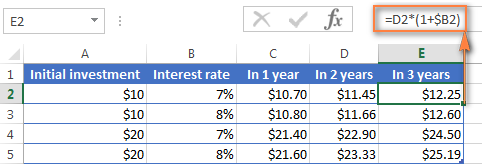Those of you who have some experience with Excel formulas have probably figured out that what the above formula actually does is multiplying the initial deposit of \$10 by 1.07 three times:

`=10*1.07*1.07*1.07=12.25043`

Round it to two decimal places and you will get the same number as you see in cell E2 in the screenshot above - \$12.25. Naturally, you can directly calculate the balance after 3 years using this formula:

`=A2*1.07*1.07*1.07`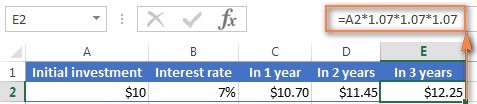#### Annual compound interest - formula 2

Another way to make an annual compound interest formula is to calculate the earned interest for each year and then add it to the initial deposit.

Assuming that your Initial deposit is in cell B1 and Annual interest rate in cell B2, the following formula works a treat:

`=B1 + B1 * \$B\$2`For the formula to work correctly, please mind the following details:

• Fix the reference to the Annual Interest Rate cell (B2 in our case) by adding the \$ sign, it should be an absolute column and absolute row, like \$B\$2.
• For Year 2 (B6) and all subsequent years, change the formula to:
Year 1 balance + Year 1 balance * Interest Rate

In this example, you'd enter the following formula in cell B6 and then copy it down to other rows, like demonstrated in the screenshot below:

`=B5 + B5 * \$B\$2`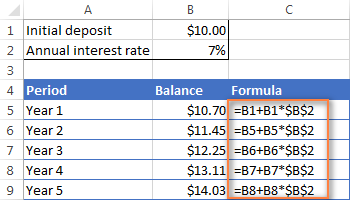To find out how much interest you actually earned with annual compounding, subtract the Initial deposit (B1) from Balance after 1 year (B5). This formula goes to C5:

`=B5-B1`

In C6, subtract Balance after 1 year from Balance after 2 years, and drag the formula down to other cells:

`=B6-B5`

You should see the earned interest growth like in the screenshot below.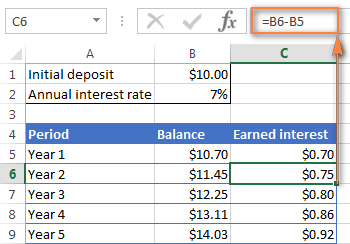The above examples do a good job illustrating the idea of compound interest, don't they? But none of the formulas is good enough to be called a universal compound interest formula for Excel. Firstly, because they do not let you specify a compounding frequency, and secondly, because you have to build an entire table rather than simply enter a certain duration and interest rate.

Well, let's take a step forward and create a universal compound interest formula for Excel that can calculate how much money you will earn with yearly, quarterly, monthly, weekly or daily compounding.

### General compound interest formula

When financial advisors analyze the impact of compound interest on an investment, they usually consider three factors that determine the future value of the investment (FV):

• PV - present value of the investment
• i - interest rate earned in each period
• n - number of periods

By knowing these components, you can use the following formula to get the future value of the investment with a certain compounded interest rate:

FV = PV * (1 + i)n

To illustrate the point better, here are a couple of quick examples.

#### Example 1: Monthly compound interest formula

Suppose, you invest \$2,000 at 8% interest rate compounded monthly and you want to know the value of your investment after 5 years.

First off, let's write down a list of components for your compound interest formula:

• PV = \$2,000
• i = 8% per year, compounded monthly (0.08/12= 006666667)
• n = 5 years x 12 months (5*12=60)

Input the above numbers in the formula, and you will get:

`= \$2,000 * (1 + 0.8/12)5x12 `

or

`= \$2,000 * 1.00666666760`

or

`= \$2,000 * 1.489845708 = \$2,979.69`

#### Example 2: Daily compound interest formula

I hope the monthly compound interest example is well understood, and now you can use the same approach for daily compounding. The initial investment, interest rate, duration and the formula are exactly the same as in the above example, only the compounding period is different:

• PV = \$2,000
• i = 8% per year, compounded daily (0.08/365 = 0.000219178)
• n = 5 years x 365 days (5*365 =1825)

Supply the above numbers into the compound interest formula, and you will get the following result:

`=\$2,000 * (1 + 0.000219178)1825 = \$2,983.52`

As you see, with daily compounding interest, the future value of the same investment is a bit higher than with monthly compounding. This is because the 8% interest rate adds interest to the principal amount each day rather than each month. As you can guess, the monthly compounding result will be higher than annual compounding.

All this is good, but what you really want is an Excel formula for compound interest, right? Just bear with me for a little longer, please. Now we are getting to the most interesting part - building your own powerful and versatile compound interest calculator in Excel.

### Compound interest formula in Excel (daily, weekly, monthly, yearly compounding)

Usually, there is more than one way to do something in Excel and a compound interest formula is not an exception :) Although Microsoft Excel provides no special function for calculating compound interest, you can use other functions to create your own compound interest calculator.

Let's start creating our Excel compound interest calculator with entering the basic factors that determine the future value of an investment in an Excel worksheet:

• initial investment (B3)
• annual interest rate (B4)
• number of compounding periods per year (B5)
• number of years (B6)

When done, your Excel sheet may look similar to this: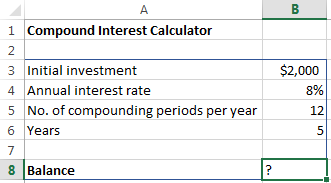All you need now is the compound interest formula to calculate the earned amount (Balance) based on the input values. The best news is that you don't have to re-invent the wheel. We will simply take the time-tested compound interest formula used by banking and other financial institutions and translate it into Excel's language.

Compound interest formula for Excel:

Initial investment * (1 + Annual interest rate / Compounding periods per year) ^ (Years * Compounding periods per year)

For the above source data, the formula takes this shape:

`=B3 * (1 + B4 /B5) ^ (B6 * B5)`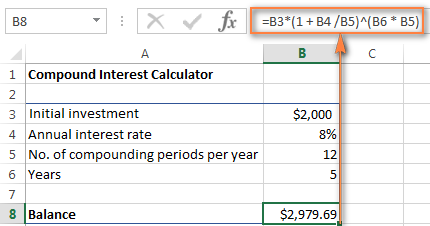The numbers look rather familiar? Yep, these are the same values and calculations that we've performed with a monthly compound interest formula, and the result proves that we did everything right!

If you want to know how much your investment will be worth at an 8% annual interest rate compounded quarterly, simply enter 4 in cell B5: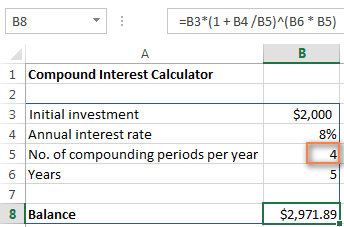To calculate the future value of your investment with semi-annual compounding, enter 2 as the Compounding periods per year value. For weekly interest rates, enter 52, this is how many weeks each year contains. If you are interested in daily compounding, enter 365, and so on.

To find the amount of earned interest, simply compute the different between the future value (balance) and the present value (initial investment). In our case, the formula in B9 is as simple as:

`=B8-B3`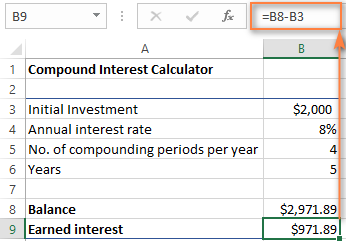As you see, we've created a truly universal compound interest calculator for Excel. Hopefully, now you have no regrets that you invested a few precious minutes in figuring out the tricky compound interest formula used by financial planners : )

### Advanced compound interest calculator for Excel

If for some reason you are not quite happy with the above approach, you can create your Excel compound interest calculator using the FV function that is available in all versions of Excel 2000 to 2019.

The FV function calculates the future value of an investment based on the input data similar to the ones we've discussed, though its syntax is a bit different:

FV(rate, nper, pmt, [pv], [type])

The detailed explanation of the arguments can be found in the Excel FV function tutorial.

In the meantime, let's build a FV formula using the same source data as in monthly compound interest example and see whether we get the same result.

As you may remember, we deposited \$2,000 for 5 years into a savings account at 8% annual interest rate compounded monthly, with no additional payments. So, our compound interest formula goes as follows:

`=FV(0.08/12, 5*12, ,-2000)`

If you need some explanation of the parameters, here you go:

• rate is 0.008/12 since you have the 8% annual interest rate compounded monthly.
• nper is 5*12, i.e. 5 years * 12 months
• pmt is left blank because we have no additional payments.
• pv is -2000 since it's an outflow and should be represented by a negative number.

Enter the above formula in an empty cell, and it will output \$2,979.69 as the result (which is perfectly inline with the result of the math calculation performed in the monthly compound interest example).

Naturally, nothing prevents you from replacing the values with cell references:

`=FV(B4/B5, B6*B5, , -B3)`

The screenshot below shows the future value of \$4,000 investment after 15 years at an annual interest rate of 7% compounded weekly: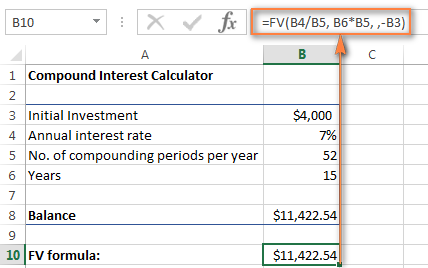To make your Excel compound interest calculator even more powerful, you can extend it with the Additional contributions option (additional payments) and modify the compound interest formula accordingly.

`=FV(B4/B5, B6*B5, -B8, -B3, B9)`

Where:

• B3 - principal investment
• B4 - annual interest rate
• B5 - the number of compounding periods per year
• B6 - the number of years to save
• B8 - additional contributions (optional)
• B9 - additional contributions type. Remember that you enter 1 if you deposit an additional amount at the beginning of the compounding period, 0 or omitted if additional payments are made at the end of the period.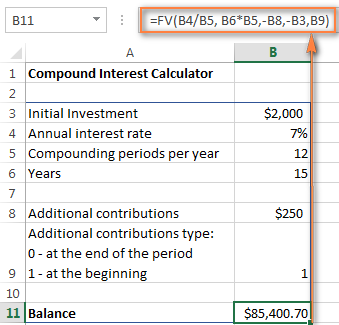If you are curious to try this advanced compound interest calculator for Excel to compute your savings, you can download it at the end of this post.

Tip. Another quick way to calculate compound interest is by doing What-If analysis with the help of Excel data table.

## Compound interest calculators online

If you prefer investing money rather than time in figuring out how to calculate compound interest in Excel, online compound interest calculators may come in handy. You can find plenty of them by entering something like "compound interest calculator" in your preferred search engine. In the meantime, let me quickly present a couple of my favorite ones.

#### Compound interest calculator by Bankrate

The key benefits of Bankrate compound interest calculator is ease-of-use and visual presentation of the results. This calculator lets you enter the savings inputs manually in boxes or by moving a slider. As you do this, the estimated total is displayed on top and immediately reflected in the graph below: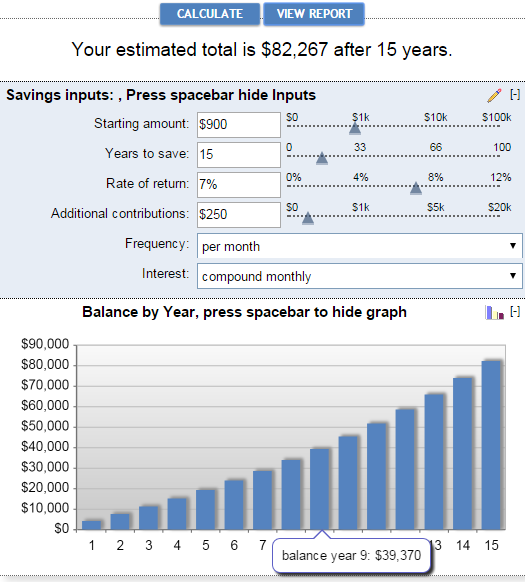Clicking the View Report button generates a "Summary Report" as well as "Savings Balance" that provides the detailed info on the amount of additional contributions, earned interest and balance for each year.

#### Compound interest calculator by Money-Zine

The online calculator from Money-Zine is much simpler compared to Bankrate's one. It asks you to specify only 3 values: the principal investment, interest rate and duration. As soon as you supply these numbers and click the Calculate button, it will show you all types of compound interest rate (daily, weekly, monthly, annual, etc.) as well as the future values with a corresponding compounding.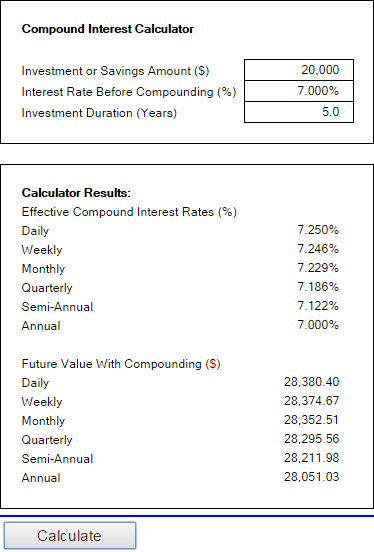#### Compound interest calculator by MoneySmart

This is a really nice online compound interest calculator run by Australian Securities and Investments Commission. It lets you input all relevant factors that determine the future value of your investment and outputs the result as a graph. By hovering over a certain bar in the graph, you can see the summary info for that particular year.This is how you calculate compound interest in Excel and outside it :) I hope at least one compound interest formula discussed in this article has proved helpful to you. Anyway, I thank you for reading and hope to see you on our blog next week!

Compound interest calculator for Excel (.xlsx file)

## You may also be interested in

1. Great article but i did not find what i was looking for. If anyone can help me out.
Develop a formula for the total amount accrued (account balance) in a CD with annual compounding after t years, if the principal = \$1,000 and the APR = 2%. Then use your formula to fill out the following table. i need help filling out the table for calculations for each of those years and the account balances for each of those years.

terms: 10 years, 20 years, 40 years, 60 years, 80 years, 100 years
Calculation:
Account balance:

2. Awesome article but, what I was looking for wasn't included here.

How do I do a compound interest on a staked token with 0.02555% interest for every 15 minutes to the initial amount acquired, on Excel

The percentage increases with 0.02555% to each balance for every 15 minutes for a period of 1 year

Please, do not use currency (\$) only just numbers because it's a token. I will multiply whatever number I get after 1 year with the current value of the token.

• Hi!
If I understand your task correctly, the following formula should work for you:

=1*(1 + 0.0002555 /1)^(365*24*4 * 1)

3. Hello Swetlana,

Thank you for this article, it helps me better understand compounding interest, but I have a somewhat different challenge as I am faced with a 4-day interest generation each week (Thursday through Sunday) and a compounding threshold. I'll exlain it further:
1. Interest 0.3%/day 4 days a week
2. Interest will only compound when it reached a minimum of \$25
3. The end-date is the moment when you'll withdraw the total amount, including compounded interest.

Question: "Ïs there a formula which takes the initial amount, the daily interest rate, a time-period in days/ weeks/month/years and gives me the total amount, so I can calculate the effective interest?"

4. Confusing and muddled explanation right from the start, muddling up interest earned and amount being invested! Surely compound interest earned is not the same as future value, which includes the princpal!
(PS - could also do with a spell check, principal has a very different meaning to principle!)

• Hello Robin,

Thank you for your feedback. You are absolutely right about "principal". Sorry for that stupid mistake, fixed.

As for the interest earned and amount being invested, I have no idea how to explain one without the other. The same is true about the future value. Nevertheless, I will try to articulate the difference clearer.

5. Hello,

i have another challenge:
i have a deposit of 5000 US\$
i have a MONTHLY Interest of 5%
compounding is calculated MONTHLY at the end of the month
i have a deposit every beginning of the month 300 US\$
it runs for 5 years

i am not able to accomplish that with exel. any formular would be nice.

best regards

paul

6. thanks

7. I am looking for a spreadsheet which can calculate interest on fixed deposit receipts for four quarters of the year, for income tax purposes.
The start date (the deposit rate) could be anything as also the maturity date. The periods of each fixed deposit and the interest rate could be different. The interest earned at the end of each quarter will be added to the principal amount and the interest calculated for the next quarter. The quarters ending will be 30 June, 30 Sept, 31 Dec of the previous year and 31 March of the subsequent year.

8. previous balance 160500.contributes 15000 every month at quarterly interest rate of 7.6%,7.25%, 7.15%,7.10% for each quarter . how can i Calculate in excel using excel formula? thanks very much.

9. How to calculate interest on rental.
July Rent is \$8000.00 per month payable on 5th of the month.
Tenant paid on 3rd August ( delayed payment after 28days)
Interest is 6% per annum
What will be the interest calculation here

10. Someone taked loan 1000\$ and he have to pay 2% interest every month.
Condition :- If he will not pay interest after 12 month he will have to pay compound interest but if he will pay some some interest amount than the date of compound interest will extend according to its given interest of months.
Example :- A person take loan 1000\$ on 30-05-20 and he have to pay 2% interest after 1 year (30-05-21) he will have to pay compound interest of 2% but if before 1 year (30-05-21) he will pay 4 months interest than the date of compound interest will extend 4 months (30-09-21).

11. Hello,
I want a formula for the following.
\$20,000 in five years time @5% annual interest
What should be the initial investment and a deposit Every month? Is it possible? or should I give a monthly deposit of @500?

Also I wish to know How do we get a simple Debt snowball formula that also calculates interest monthly as well as annually
Kind Regards
Constantine

12. Can any one help me to write excell formula and calculate FV for the following.
Beginning Investment = 10774716
Interest Rate = 10% per annum
No of Payments = 5
Monthly Contributions = 83816
Regards

13. Hi Svetlana - Thank you for your great article. I believe its the most comprehensive on the internet for calculating CI using Excel. However, the compound interest problem I'm working on is a bit tricky. That's because it has 2 tricks, the interest rate is changing each month and also because the problem is asking for the interest amount at the end of a specific period of which the last date is actually at the middle of a month, not at the end of it, meaning the last 15 days of the period is not eligible for compounded interest.

The problem says find the excel formula that can calculate the monthly compounding interest on a US\$1000 investment at the end of 1905 days using the published 1 Month USD Libor rate that is published at the beginning of each month by theice.com starting January 1st, 2014. The problem assumes the year equal to 360 days. So, 1905 days is 5 years, 3 months and 15 days!!

So, in effort to resolve the 15 days trick first, I assumed the interest is fixed thru out the period and I reached the following concept formula that calculates the monthly compounding interest at the end of any given period whether the period ends at the end of a month or at any day within a month:
(P*(1 + r/360*30)^m - P) + (P*(1 + r/360*30)^(m-1))*r/360*d
where:
P = the principal amount (the \$1000)
r = the fixed annual interest rate
m = the count of full months within the given investment period
d = the count of days at the end of the period that didn’t complete a full month yet

And then I reached the following universal excel formula to apply the above concept formula:
(A1*(1+A2/360*30)^INT(A3/30))-A1+IF(INT(A3/30)=A3/30,0,(A1*(1+A2/360*30)^(INT(A3/30)-1))*(A2/360*((A3/30-INT(A3/30))*30)))
where:
A1 = The principal amount (the \$1000)
A2 = The annual interest rate
A3 = The investment period

But now, I am stuck trying to incorporate the monthly changing interest rate in the formula, can you help me? :D

Thank you!

14. how to calculate per day interest rate in exel example i deposite 5000 daily and i got 2000000 after 365 days

15. please explain me how to calculate per day interest rate in excel

16. Hi everybody

I need the formula to calculate the compound interest between to specific dates.
Thank you.

17. if Payments of \$ 670 are being made at the end of each month for 5 years at an interest of 8% compounded monthly. Calculate the Present Value

18. give me calculation for loan repayment using compound interest compounded yearly.

19. My request,
I have deposited fixed deposits on cummulative basis(drawn on maturity) in various banks, various rate of interest, starting and ending dates. All these deposits in months i.e. 15,22,27, 37 months like this. Interest will be calculated quarterly basis. I want excel correct calculation or excel template, along with finanical year interest between 1st April year to 31st March next year(say 01-04-2018 to 31-03-2019) for income tax purpose. And if financial year interest exceeds INR 50000/- otherwise no tds deducted. Ex: start date/ end date/ rate / Deposits in months / invest amt / maturity amt / total interest/ financial year interest/ Tax deducted at source at 10% on FY Interest. I hope you understand my request and send an excel statement with formula incorporated to my email id.
GOPINATH

20. HI, need some help. I am creating an Excel spreadsheet that demonstrates the compound results on retirement investments over time. It compares several individuals, all invest funds annually but for different time periods. For example, one begins at age 20, contributes each year for 10 years then stops contributing but all funds compound annually for 45 years. Another contributes annually for 35 years but begins after the first individual stops making contributions. A third makes 45 years of contributions. All funds compound annually at 8%. Struggling with the formulas.

21. Hello,
I have a set amount of \$1.75 million that compounds daily at a rate of 8% per annum. I am having difficulty showing each month's principal and interest accrued to the end of each month. I know there has to be a Excel formula to do this for each month on the calendar reflecting the different number of days in each month, but it just isn't coming to me. I sure could use your help. Thanks. Jim

22. I am trying to calculate interest on back pay. The interest is compounded daily and there is new principle added every two weeks (not to mention the interest rate changed over the relevant period) is there a formula for this? For example, employee A was supposed to be paid \$2000 every two weeks, he is due back pay with 4% interest compounded daily for say 6 months. How would I calculate this?

23. I have a loan that I will make monthly payment,but interest compound daily. How can I show it in Excel?
Borrow amount: 10,800.00
Annually Rate: 6.75%
Date taken 06/06/18
First payment: 07/01/18

Thanks,

24. Dear all,

I like to know the excel formula for fixed deposit which compounding in certain period when entry and exit dates are provided.

Example: I have deposited \$.2,000 on 11th Oct 2018 @ annual rate of 10% with quarterly compounding. What will be my future value on 8th Sept 2019.

We can get the future value as \$.2,188.75 at end of period as worked out below.
But If I have series of deposit to hundreds of people how I can get in once coloum with future value

Say : Start Date End Date Principal Amount Interest rate Result
11/10/18 08/09/19 2000 10% Formula

Start Period Start Amt. End Period Interest End Amt. Days
11/10/18 2000.00000 10/01/19 50.4109589 2050.410959 92
11/01/19 2050.410959 10/04/19 50.55807844 2100.969037 90
11/04/19 2100.969037 10/07/19 52.38032394 2153.349361 91
11/07/19 2153.349361 08/09/19 35.39752375 2188.746885 60

Can any body help me in this task.

Thanking you

25. Hi Maria, I was just wondering if one can calculate compound interest at a certain interest rate, compounded quarterly, but with the additional payment being every month and not every quarter. Any help would be appreciated!

26. In the line that begins:

= \$2,000 * (1 + 0.8/12)5x12

I think you mean:

= \$2,000 * (1 + 0.08/12)5x12

As 0.8 would be an interest rate of 80%

27. I am trying to use the Excel FV to calculate the compound interest of a series of annual payments, compounded quarterly. I can divide the annual interest rate by 4 and multiply the terms to num of years * 4. This does not give the same as done long way in Excel. The long way is calculate quarterly compounded interest each year and then add annual payment. This is compounded the next year and so forth. Is this possible to be done using Excel functions?

28. how to calculate between two dates compound interest
Ex 25.02.2017 loan taken
PV 100000 Int rate 12%

29. I need to find out what is currently owed on a past due debt that is incurring interest. I have several debts owed to me that I need to track. For example, one debt from July 01, 2015 with a principle of \$267.27 that is accruing interest of 12% per annum. from August 01, 2015 through today's date. A second debt from August 01, 2015 with a principle of \$623.12 that is accruing interest of 12% per annum. through today's date. A third debt from January 01, 2017 with a principle of \$6500 and an interest rate of 18% through today's date.

I need an excel spreadsheet that can show the court the amount currently owed on each debt seperatly and how that amount has grown.

I hope that is clear.

30. I have a loan for 25,000 at 7% interest with a total payback of 32,250 this loan is paid back on a daily basis for 12 months except for holidays and weekends. The total number of payments are 252. The daily payment is 128.00. How do I do an amortization table?

Thank you

31. Hi there,

I was wondering if you could please assist with this following question:

What is the compound interest of any amount invested at 6%, if the interest is capitalized quarterly?

Best,

Emmanuel.

32. .please calculate the compounding interest of 4000 bd after 3 years of paying at 130 bd per month

33. I have to create a spreadsheet of a principal amount owing, compounded daily, at interest rates that change every 3 months, with occasional payments to reduce principal, over a period of 8 years. Can you help me with my formula? I have a list of the various interest rates.

34. Hi

How do you write excel formula to give below dates in a column
In this example I've chose 7 days
Each cell down is +7 days from the previous cell, except when it gets less then 7 days then it is the last day of the month and next cell after the the end of month is +7 days from the previous 2 cells

14/7/17 (start date, can be any date of the month)
21/7/17
28/7/17
31/7/17 (Here needs to be end of month)
4/8/17 (Here needs to be 28/7/17 + 7days)
11/8/17
18/8/17
...and so on

Thank you

• Hi, Eddie,

The best, quicker and easier, option would be a macro or VBA code. You can ask around Mr. Excel forum for them.

If you'd like to use a formula, here's an example:

1. Format column A as a date (Home tab > Number format)
2. Input start date to A1 (14/7/17)
3. Put the formula below to A2 cell:
=IF(\$A1=EOMONTH(\$A1,0),IFERROR(IF((OFFSET(\$A1,-1,0)+7)=EOMONTH(\$A1,0),(\$A1+7),(OFFSET(\$A1,-1,0)+7)),(\$A1+7)),IF(MONTH(\$A1)=MONTH(\$A1+7),(\$A1+7),EOMONTH(\$A1,0)))
4. Copy it down the column by drag-and-dropping

Hope this helps!

• Hi Natalia.

Fantastic! Thank u so much for the formula and tip.
Wishising u all the best.
Regards
Eddie

• Hi Natalia,

Can you help me with the next stage of the formulas...

=IF(\$A1=EOMONTH(\$A1,0),IFERROR(IF((OFFSET(\$A1,-1,0)+7)=EOMONTH(\$A1,0),(\$A1+7),(OFFSET(\$A1,-1,0)+7)),(\$A1+7)),IF(MONTH(\$A1)=MONTH(\$A1+7),(\$A1+7),EOMONTH(\$A1,0)))

be changed so that if the end of the month falls on a weekend then it gives the date of the last working business day. Then rule continues as before.

Current formula gives
15/9/17
22/9/17
29/9/17
30/9/17 since this is Saturday (weekend)
6/10/17

Then result should be
15/9/17
22/9/17
29/9/17
29/9/17 repeat same date as previous cell only if it falls on a weekend
6/10/17 continue with the standard rule (29/9/17+7 days)
13/10/17
.... and so on

Next stage formulas:
Also how can your 1st formula and your 2nd formula above be rewritten so rather than 7 day increments, how can I change the interval date to any number so that it still follows the above two formula rules.

Your new formula ......(based on any incremental number)
Your new formula ......(based on any incremental number and weekend rule)

Thank you so much for your help

• Eddie,

we've adjusted the formula for you that includes the weekend rule:
=IF(\$A1=EOMONTH(\$A1,0),IFERROR(IF((OFFSET(\$A1,-1,0)+7)=EOMONTH(\$A1,0),(\$A1+7),(OFFSET(\$A1,-1,0)+7)),(\$A1+7)),IF(MONTH(\$A1)=MONTH(\$A1+7),(\$A1+7),IFERROR(IF(OFFSET(\$A1,-1,0)=\$A1,(\$A1+7),IF(WEEKDAY(EOMONTH(\$A1,0),2)=6,EOMONTH(\$A1,0)-1,IF(WEEKDAY(EOMONTH(\$A1,0),2)=7,EOMONTH(\$A1,0)-2,EOMONTH(\$A1,0)))),(\$A1+7))))

Also, for the case when you want to use some incremental number (let's say it's placed to B1 cell), we have the following:
=IF(\$A1=EOMONTH(\$A1,0),IFERROR(IF((OFFSET(\$A1,-1,0)+\$B\$1)=EOMONTH(\$A1,0),(\$A1+\$B\$1),(OFFSET(\$A1,-1,0)+\$B\$1)),(\$A1+\$B\$1)),IF(MONTH(\$A1)=MONTH(\$A1+\$B\$1),(\$A1+\$B\$1),EOMONTH(\$A1,0)))

But when you want to use both, incremental number and weekend rule, the formula becomes too complex and long. You need to use a VBA code or a macro.

• Thank u soo much Natalia

• You're welcome!

• can explain the same